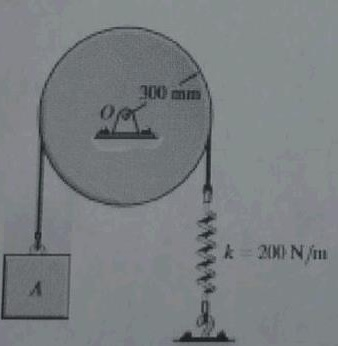# The 20 kg disk is pinned at its mass centre O, and supports the 4 kg block A. If the belt which...

## Question:

The 20 kg disk is pinned at its mass centre O, and supports the 4 kg block A. If the belt which passes over the disk is not allowed to slip at its contacting surface, determine the natural period of vibration of the system without damping (free vibration). Find the equation of motion.## Frequency

The particle having simple harmonic motion vibrates along its axis. It repeats its cycle from its mean position as number of oscillation per second. It is measured in hertz. It is reciprocal to that of the time period.

Given data

• The value of the mass of the disk is {eq}{m_{disk}} = 20\;{\rm{kg}} {/eq}
• The value of the mass of the block A is {eq}{m_{blcok\;A}} =...

Become a Study.com member to unlock this answer! Create your accountVibrational Spectroscopy: Definition & Types

from Physical Science: Help and Review

Chapter 10 / Lesson 18
23K

Support